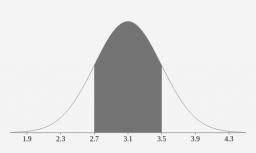# Distribution 6282

The waiting time in the buffet is governed by the normal distribution with a mean value of 130 seconds and a variance of 400. What is the probability that someone will wait less than a minute and a half?

p =  2.28 %

### Step-by-step explanation:Did you find an error or inaccuracy? Feel free to write us. Thank you!

Tips for related online calculators
Looking for help with calculating arithmetic mean?
Looking for a statistical calculator?
Looking for a standard deviation calculator?
Would you like to compute the count of combinations?

#### Grade of the word problem:

We encourage you to watch this tutorial video on this math problem: